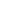# Free Class 7 Addition and subtraction of algebraic expressions Worksheets

Download free printable Addition and subtraction of algebraic expressions Worksheets to practice. With thousands of questions available, you can generate as many Addition and subtraction of algebraic expressions Worksheets as you want.

## Sample Class 7 Addition and subtraction of algebraic expressions Worksheet Questions

1.

Fill in the blanks: We get ________ when x - y is subtracted from 2x - y.

2.

Fill in the blanks: The sum of ( -6x + y2) and (-4x - y2) is _______.

3.

Fill in the blanks: We get _______ when we subtract (x - 2x2) from 1

4.

Fill in the blanks: When -4x2 is subtracted from 0 we get ______.

5.

What must be added to 12x3 - 4x2 + 3x + 7 to make the sum x3 + 2x2 - 3x + 2

6.

How much is x2 - 2xy + 3y2 less than 2x2 - 3y2 + xy

7.

How much is x - 2y + 32 greater than 3x + 5y - 7

8.

What should be subtracted from x2 - xy + y2 - x + y + 3 to obtain -x2 + 3y2 - 4xy + 1

9.

Subtract the sum of 13x - 4y + 7z and -6z + 6x + 3y from the sum of 6x - 4y - 4z and 2x + 4y - 7

10.

From the sum of 3x2 - 5x + 2 and -5x2 - 3x + 9 subtract 4x2 - 3x + 9

Worksheets by UrbanPro

Our worksheets are designed to help students explore various topics, practice skills and enrich their subject knowledge, to improve their academic performance. Designed by Experts who have extensive experience and expertise in teaching a subject, these worksheets will improve your child's problem-solving skills and subject knowledge in a fun and interactive manner.
Check out our free customized worksheets across school boards, grades, subjects and levels of subject knowledge. You can download, print and share these worksheets with anyone, anywhere, anytime!

Get a custom worksheet to practice!

Select your topic & see the magic.

subjectSelect Chapter(s)

Chapters & Subtopics